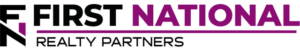# Understanding the Internal Rate of Return

### Key Takeaways

• Prior to making a commercial real estate investment, it is important for individuals to estimate the return potential of the deal.  One of the ways they do this is by calculating a metric known as the Internal Rate of Return.
• The Internal Rate of Return is the discount rate that sets the Net Present Value of a property’s future cash flows equal to 0.  The mathematical formula to calculate it can be complex.  Instead the “IRR” function can be used in a spreadsheet program.
• To obtain the cash flows needed to calculate the Internal Rate of Return, it is first necessary to create a proforma projection.  Income less operating expenses and debt service equals Cash Available for Distribution, which is the key input in the IRR calculation.
• While IRR is an impactful metric, it has its limitations.  It can be skewed by high cash flows that occur early in the investment period and it doesn’t provide an indication of the absolute return on an investment.
• To account for its limitations, other metrics are considered along with Internal Rate of Return, most notable the Equity Multiple.

Fundamentally, the idea of making a commercial real estate investment is a simple one.  An individual contributes a certain amount of capital towards the initial cost of an investment property.  In doing so, they expect that they will receive their initial investment back, plus a return over time.  Prior to making an investment decision or when comparing investment options, it is only logical that this individual would want to know how much of a return they could potentially earn to decide whether or not it is worth the risk.

One of the ways that commercial real estate returns are measured is through a metric called the Internal Rate of Return.

## What is Internal Rate of Return?

The internal rate of return is the discount rate that sets the net present value (NPV) of all future cash flows equal to zero and it is often used as a proxy for the annual rate of return/interest rate that is earned on a projected series of cash flows.  The word “internal” in the name refers to the fact that external factors such as the risk free rate, inflation, and the cost of capital are not considered in its calculation.

The formula used to calculate the Internal Rate of Return is rooted in the concept of the “time value of money”, which is the idea that a dollar received today is worth more than a dollar received in a future time period because of its ability to be continually reinvested and earn interest.  To account for this concept, the IRR formula can be quite complex so it can be easier to use an online financial calculator or the IRR function in a spreadsheet program like Microsoft Excel.

To illustrate how internal rate of return works, an example is helpful (NOTE:  It is acknowledged that there are commonly used IRR variations like Modified Internal Rate of Return (MIRR) or XIRR, but these are not considered as part of this article).

## Calculating IRR – An Example

IRR is often calculated prior to making an investment so it relies upon the cash flow projections contained in a property proforma.  Assume that an investor is considering purchasing a property for \$1.25MM and they have projected the following proforma cash flows for it: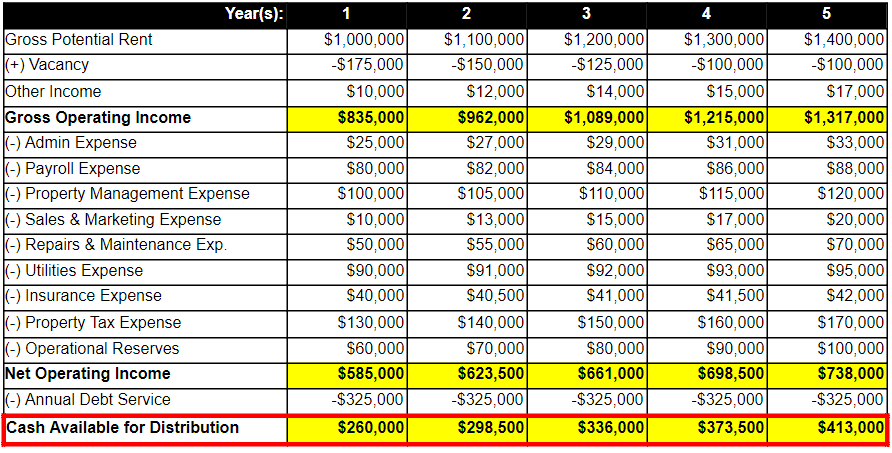In the creation of the proforma, the investor estimates what future income and expenses will be based on the property’s past performance and comparable assets in the market.  Income less expenses is known as Net Operating Income and, once debt service is subtracted, a figure known as Cash Available for Distribution is left.  It represents the money that is available to be distributed to investors and, along with the purchase price,  these are the inputs in the internal rate of return calculation.  The cash flows can be rearranged to look like this: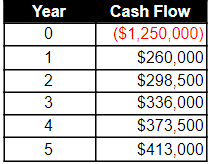The cash flow in year 0 represents the purchase price (the cash outflow) of the potential investment and the subsequent positive cash flows (cash inflows) represent those calculated in the proforma projection.  These can be put into the IRR spreadsheet function as follows:

Internal Rate of Return = IRR(-1,250,000, 260,000, 298,500, 336,000, 373,500, 413,000)

The result of 9.91% suggests that this is the annual return on investment that an investor could expect to earn if the actual cash flows track closely to those projected in the proforma.  While this is a promising return, there are some internal rate of return limitations that potential investors should be aware of.

## Internal Rate of Return Limitations

Again, the IRR calculation is rooted in the time value of money concept, which presents two key limitations in the resulting estimate.

First, the time value of money concept means that more consideration is given to larger cash flows that occur earlier in the period.  To prove this point, the cash flows in years 1 and 5 from the table above are swapped as follows: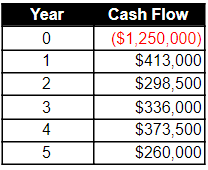The result is a higher internal rate of return of 11.35% because the larger cash flow of \$413,000 occurs in year 1 and can be reinvested to earn interest for 4 years.  So, potential investors should be aware of the order of cash flows and how they contribute to the projected IRR.

The other limitation is that internal rate of return does not provide any indication of the absolute return on an investment.  For example, an initial investment of \$1.25MM that returns \$1.3MM (original \$1.25MM plus \$50M) in one month has an IRR of ~60.10%, which seems fantastic.  In reality, the investor has earned \$50M on their investment, which is a relatively small sum given the initial investment.  For this reason, internal rate of return is commonly used alongside another metric, the Equity Multiple, to provide a more clear picture of an investment’s potential return.

## Interested In Learning More?

First National Realty Partners is one of the country’s leading private equity commercial real estate investment firms. With an intentional focus on finding world-class, multi-tenanted assets – including middle-market service-oriented retail shopping centers – well below intrinsic value, we seek to create superior long-term, risk-adjusted returns for our investors while creating strong economic assets for the communities we invest in.

When evaluating an investment opportunity, we review both the Internal Rate of Return and Equity Multiple as an indication of potential profitability and communicate them as part of the information provided to potential investors.

Get instant access to all of our current and past commercial real estate deals.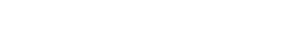## Subscribe Now

Get the latest news on real estate

FNRP Spotlight Series

1031 Exchange

Acquisitions

## Get More From FNRP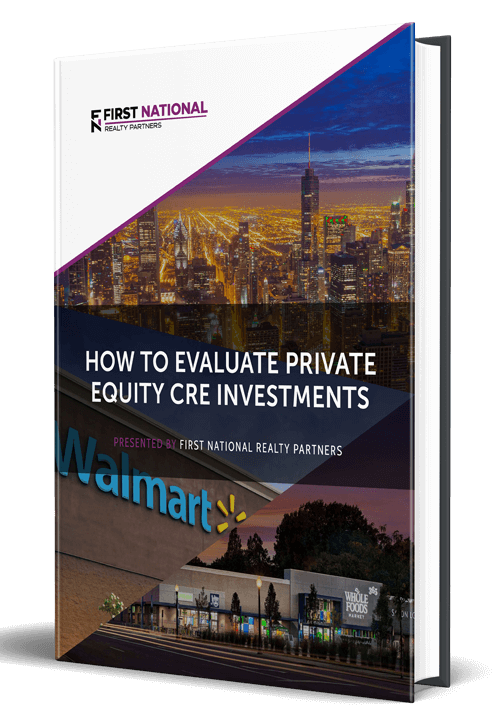## How to Evaluate Private Equity CRE Investments## How to Complete a 1031 Exchange with a Private Equity Sponsor